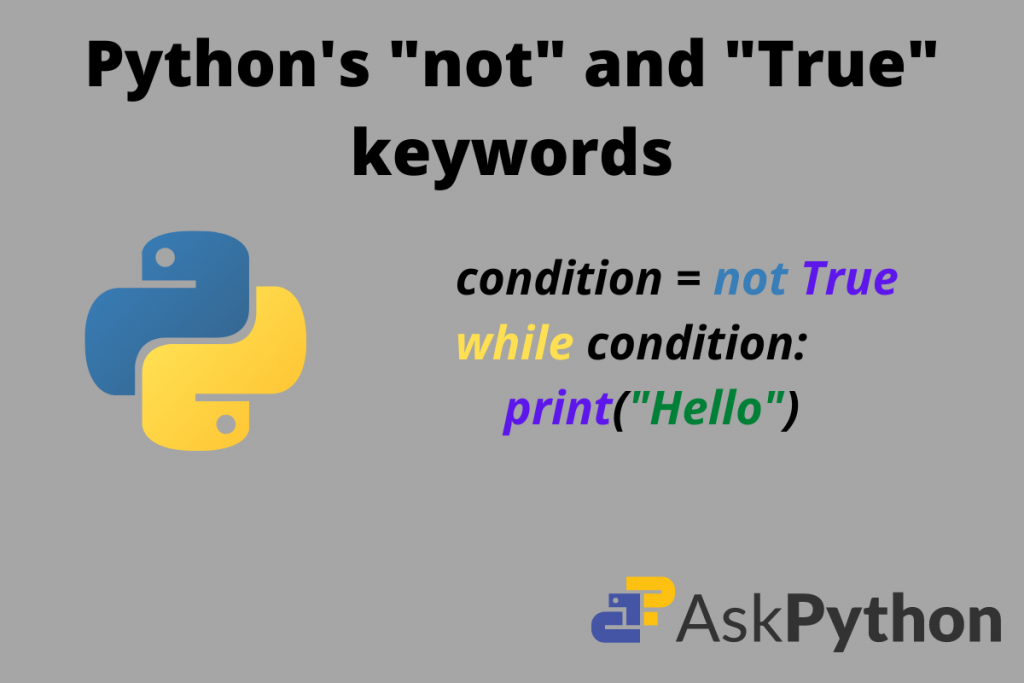# What is “not True” in Python?In this article, we all going to talk about the not True concept in Python. Generally, it is not a boring lecture rather we are just polishing our basics.

## Operators and keywords in Python

Python has a large set of operators. That is why, unlike any other programming language, it relatively has lesser complex syntax. Here is a list of operators in Python:

1. + : Plus
2. – : Minus
3. = : assignment operator
4. == : equal to operator
5. != : not equal to
6. <= : less than or equal to
7. >= : greater tha n or equal to
8. % : modulus
9. // : floor division
10. -= : decrement
11. += : increment
12. /= : divide
13. %= : modulus

These support arithmetic operations but most importantly we have some other ones for logical operations:

1. & : and
2. | : or
3. not

Also, take a look at the boolean operations:

1. True
2. False

We can use logical operators either like keywords or as it is. But, in Python, we do not have any operator for not or complement. Obviously, there is “!=” but it is suitable for small operations. For complex manipulations, we can make things simpler using the “not” keyword.

## Significance of “not” in Python

This example is alone sufficient to prove how “not” is useful:

Predict whether the while loop will run or not

Code:

```condition = not True
while(condition):
print("Hello world")
```

The code will not run. The while loop iterates the code if and only if the condition inside its parenthesis is True. Here the condition is not True means it is False. If you run the small snippet in IDLE this will also give output as False.

```>>> not True
```
```False
```

So, this is the significance of a not operator.

## Significance of “True” in Python

True is a boolean operator in Python. The significance is that one can set flags, run a loop and do many more things with it. Let us see an example:

Print “hello” n number of times on the screen.

```while True:
print("Hello")
```

Output:

```Hello
Hello
Hello
Hello
...
...
Runtime Error occurred
```

The last message is “Runtime Error occurred”. This means that when we run the loop infinitely using True and there is no loop control statement then it goes on executing that piece of code n number of times. This is something to note.

## Using not and True together

Here we will build a code that checks each number and prints out whether it is prime or not.

```num = int(input("Enter a number: "))
isPrime = not True
num_sqrt = int(num**0.5)

if(num > 1):
for i in range(2, num_sqrt + 1):
if (num % i == 0):
isPrime = True
break
if (isPrime == (not True)):
print("%d is prime" %(num))
else:
print("%d is composite" %(num))
else:
print("%d is composite" %(num))
```

Output:

```>>> Enter a number: 39
39 is not prime

>> Enter a number: 17
17 is prime
```

Explanation:

1. First, take the input for num.
2. Then set a variable of name isPrime. This is just an indicator that initially indicates a value that is not True.
3. Then we take the square root of the number.
4. Then we put a condition that if the number is greater than 1. It runs a loop that iterates from 2 to (square root of number +1).
5. Then for each iteration, we check whether the number is divisible by itself. If it is then the indicator isPrime is set to True. This means the number is prime.
6. If this is not the case then number is composite.

Here not True works together as False. The main motive to explain is is that we can use it in place of False.

## Conclusion

In this way, we can use the concept of not True together So, hope this article is helpful and we came to know that we can flexibly use concepts of Python for our benefit.